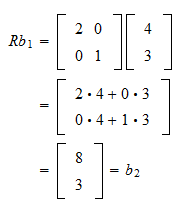StatLect

# Equivalent systems of equations

Two systems of linear equations are equivalent if and only if they have the same set of solutions. In other words, two systems are equivalent if and only if every solution of one of them is also a solution of the other.

All the main methods used to solve linear systems are based on the same principle: given a system, we transform it into an equivalent system that is easier to solve; then, its solution is also the solution of the original system.## Definition

Remember that a system ofequations inunknowns can be written in matrix form aswhereis thematrix of coefficients of the system,is thevector of constants andis thevector of unknowns.

Definition Letandbe two linear systems, both having anvector of unknowns. The two systems are equivalent if and only if they have the same solutions, that is, if and only if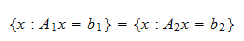## How to transform a system into an equivalent one

A system of equations can be transformed into an equivalent one by pre-multiplying both sides of its matrix form by an invertible matrix.

Proposition The system ofequations inunknownsis equivalent to the systemfor any invertiblematrix.

Proof

Supposesolves the systemthat is,andare the same vector. Clearly, if we pre-multiply the same vector by the same matrix, we obtain the same result. As a consequence,which is the same asThus, we have proved that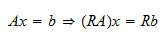Now, supposesolves the systemWe have assumed thatis invertible, so that its inverseexists. We can pre-multiply both sides of the last equation byand obtainor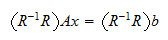Since, we haveThus, we have proved that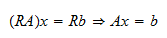which, together with the implication derived previously, gives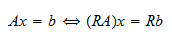that is,is a solution of one of the two systems if and only if it is a solution of the other one. In other words, the two systems are equivalent.

Example Consider the system of two equations in two unknownsThe system can be written in matrix form aswhere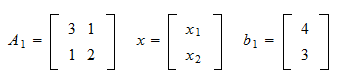If we multiply the first equation byand leave the second equation unchanged, we obtain a new systemThe matrix form of the new system iswhereThe new system is equivalent to the original one because the same result can be achieved by pre-multiplying the matrix form of the original system by the invertible matrixIn fact,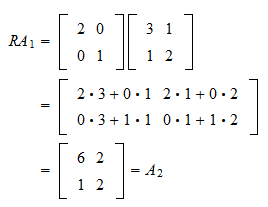and